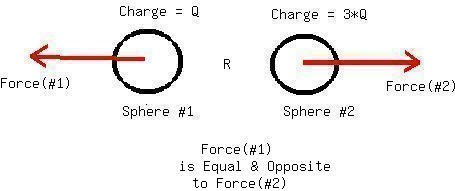Question# Two uniformly charged spheres are firmly fastened to andelectrically insulated from frictionless pucks on an airtable. The charge on sphere 2 is three

Other
ANSWEREDTwo uniformly charged spheres are firmly fastened to andelectrically insulated from frictionless pucks on an airtable. The charge on sphere 2 is three times the charge onsphere 1. Draw the force diagram that correctly showsthe magnitude and direction of the electrostatic forces. Explain your reasoning.2021-05-08Let "R" be distance between Sphere centers. Then vector Force "$$\displaystyle{F}_{{1}}$$" on Sphere #1 is equal & opposite to vector Force "$$\displaystyle{F}_{{2}}$$" on Sphere #2:
$$\displaystyle{F}_{{1}}=-{F}_{{2}}$$
The Spheres REPEL each otherbecause they each contain the SAME-SIGN charge. Thus, using Coulomb's Law:
$$\displaystyle\text{Magnitude }\ {F}_{{1}}=\text{Magnitude }\ {F}_{{2}}={\frac{{{1}}}{{{4}\cdot\pi\cdot\epsilon_{{0}}}}}\cdot{Q}\cdot{\frac{{{3}\cdot{Q}}}{{{R}^{{2}}}}}$$
Direction $$\displaystyle{F}_{{1}}$$=OPPOSITE Direction $$\displaystyle{F}_{{2}}$$
{Sphere #1 &Sphere #2 REPEL Each Other}